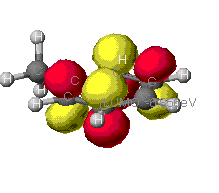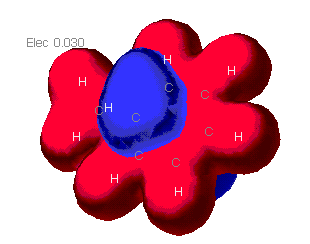# Theoretical Calculations of Properties of Toluene

by Dustyn Sawall

### Types of calculations performed

In general, Molecular orbitals (MO) are combinations of Atomic orbitals (AO). Atomic orbitals are actually mathematical expressions representing where a electron in a given quantum state can be found. When AO's are added together, they are done so in a way to minimize the energy of the system. This is done by some contributions of AO's being greater than others. The mathematical formula for this simplifies into three type of factors, the energy of the original AO, the energy saved by forming the bond and an overlap factor dependent on multiple interactions between electrons that need to be evaluated at the same time.

These equations rapidly become huge. To do them for all of the overlap considerations takes considerable time and computing power. Even then, they don't all work out. These are known as ab initio calculations. To improve workability of the mathematics, some modifications can be made. Generally, the more of the overlap integrals you use, the better your calc, but that is a trade off with if they can be done at all.

In the programs used for this page, three different levels of theory are analyzed. In the extended Huckel theory, it is assumed that only nearest neighbors interact. Zindo level of theory uses intermediate neglect of the overlap intergrals. It works using some empirical data from ab initio calculations to optimize its MO's. MOPAC level of theory uses a moderate neglect of these intergrals and uses empirical data from thermodynamics to improve it's theory. MOPAC is the most precise of the programs used because it takes into account more of the intergrals in question.

### Results of geometry optimization at various levels of theory

Initially, only a Molecular Mechanics program was used. This program treats the atoms as classical harmonic oscillator. The bond lengths and angles are calculated from rotational and vibrational spectroscopy results. The computer has tables of bond lengths, the computer then uses standard values from these tables to optimize the molecules. The molecule is designed so as to minimize the stress between atoms. This is the program used for the extended huckel level of theory. Zindo and MOPAC have geometry optimizations available to them from quantum theory to further optimize the molecules. In theory, the Mopac calc should be best. If the bond lengths and angles were examined in detail, there would be slight differences, but as can be seen from the pictures below, they are slight.

 Extended Huckel MOPAC ZINDO

### The molecular orbitals that provide the primary bonding

Here are a few of the molecular orbitals for toluene. This is only two of the orbitals that provide bonding for the molecule, there are several others. As can be seen, the MO's can cover large portions of the molecule.This is an example of a sigma bond from the carbon atoms throughout the benzene ring.This is one veiw of the pi bond formed from the p orbitals in the carbons of the benzene ring.This is simply another veiw of the pi bond. Notice how the orbital extends in to the co-planar H's of the methyl group.

### The highest occupied (HOMO) and lowest unoccupied (LUMO) molecular orbitals

These are the orbitals that are most involved in chemical reactions since they can most easily lose or gain electrons.Extended HuckelMOPAC and ZINDOAs can be seen from these diagrams, the extended Huckel theory and the other two often differ. This is because of the different assumptions each make. In general the MOPAC level of theory is superior. These show the orbitals that are at the Highest Occupied MO, that is the MO that is occupied at an elevated energy level. The red/yellow is the Lowest Unoccupied MO. There are no electrons in these MO's as they are at such an elevated energy level.

### Electron density/distribution mapsMOPAC
This is diagram showing the probability of finding an electron in the given areas of map, the warmer the color, the more likely that an electron could be found there.

### Charge distribution mapsMOPAC

These maps show the relative distribution of positive and negative charge on the molecules. The red indicates a positive charge and blue is for a negative charge. As can be seen here, the molecule mostly presents a positive charge uniformally. The only negative areas are outside of the plane of the molecule. It would be predicted that a partial negative charge would be found above and below the ring. A partial positve charge could be predicted near the methyl group.

### Calculated dipole moments

 Method used Dipole Moment Extended Huckel .733 Mopac .262 Zindo .525 CRC .375